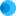Intuit HelpIntuit

# Calculating MACRS depreciation in ProConnect Tax

SOLVEDby IntuitProConnect Tax1Updated July 27, 2021

This article describes how ProConnect Tax calculates straight line and declining balance depreciation amounts using the Modified Accelerated Cost Recovery System (MACRS).

## Straight line depreciation method

ProConnect Tax calculates straight-line depreciation by taking the basis and dividing it by the life of the asset.

So, for a 7-year depreciable asset, 0.14 of the basis will be allowed in depreciation each year until it's fully depreciated.The program will calculate other limiting factors, such as business use percentage, before applying auto limits, if applicable.

## Declining balance depreciation method

The percentage depreciation is based on a 200% declining balance calculation:

Depreciation = ( Previous Year Non-Depreciated Basis / Life of Asset ) x 2

When straight-line depreciation for the remaining years (taking into consideration that year one is a half-year) would equal or exceed the declining balance depreciation, the depreciation shifts to straight-line for all remaining years.

For example, an asset with a 5-year life and purchase price of \$5,000 would depreciate as:

• Year 1 (half year): (\$5,000 / 5) x 2 = \$1,o00
• Year 2: (\$4,000 / 5) x 2 = \$1,600
• Year 3: (\$2,400 / 5) x 2 = \$960
• Year 4: (\$1,440 / 5) x 2 = \$576 (straight-line calculation beings here)
• Year 5: \$1,440 / 2.5 = \$576
• Year 6 (half year): (\$1,440 / 2.5) x 2 = \$288
• \$1,000 + \$1,600 + \$960 + \$576 + \$576 + \$288 = \$5,000

The first and last year's depreciation are half-year calculations, so you must divide the allowable depreciation by 2 for both years.

### Related topics: